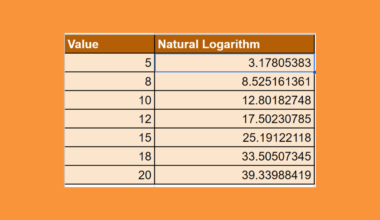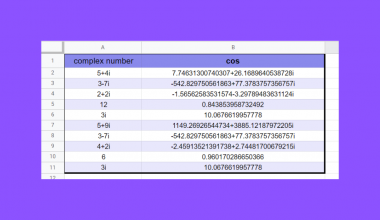# How to Use REDUCE Function in Google Sheets

This guide will explain how to use `REDUCE`function in Google Sheets.

When we want to reduce an array to an accumulated result using the `LAMBDA` function for each value, we can utilize the `REDUCE` function in Google Sheets.

The rules for using the `REDUCE` function in Google Sheets are the following:

• The `REDUCE` function is used to turn a given array into a single value by applying a custom `LAMBDA` function to each value in the given array.
• The `LAMBDA` function must have exactly 2 name arguments. Otherwise, a #N/A error is returned.
• The formula_expression argument must use those 2 names inputted in the name argument

Google Sheets released the `LAMBDA` function alongside with several Lambda helper functions (LHFs) that allow us to elevate the `LAMBDA` function and perform complex array operations.

We can use the `LAMBDA` function as a standalone but it becomes more powerful when we use it together with Lambda helper functions.

Some Lambda helper functions are the `MAP`function, `REDUCE` function, `BYCOL` function, `BYROW` function, `SCAN` function, and `MAKEARRAY` function. We will focus on using the `REDUCE` function with the `LAMBDA` function.

In this guide, we will provide a step-by-step tutorial on how to use the `REDUCE` function in Google Sheets. Additionally, we will explore the syntax and a real example of using the function.

Great! Let’s dive right in.

## The Anatomy of the REDUCE Function

The syntax or the way we write the `REDUCE` function is as follows:

`=REDUCE(initial_value,array_or_range,LAMBDA)`
• = the equal sign is how we begin any function in Google Sheets.
• REDUCE() is our `REDUCE` function. This function will reduce an array to an accumulated value and then return the total value in the accumulator.
• initial_value is a required argument. This argument refers to the initial accumulator value.
• array_or_range is another required argument. This is the array or range we want to reduce.
• LAMBDA is also a required argument. This refers to the `LAMBDA` formula we want to apply to each value in the array_or_range

## The Anatomy of the LAMBDA Function

The syntax or the way we write the `LAMBDA` function when using it with the `REDUCE` function is as follows:

`=LAMBDA(name1,name2,formula_expression)`
• = the equal sign is how we activate any function in Google Sheets.
• LAMBDA() is our `LAMBDA` function. This function is used to create and return a custom function with a set of names and a formula that uses them.
• name1 is a required argument. This refers to the name we use for the accumulator value.
• name2 is another required argument. This refers to the calculation applied to each value in the given array or range.
• formula_expression is a required argument. This refers to the formula we want to calculate or execute. We need to use the names we inputted in the name argument.

Note: There is a different syntax when using the `LAMBDA` function as a standalone. Please refer to this guide to learn about the complete anatomy of the `LAMBDA` function.

## Preparing the LAMBDA Function

Before we can use the `REDUCE` function, we need first to prepare our `LAMBDA` function. Let’s say we want to calculate the cumulative sales position for December 2022 without calculating the other months in the year.

The data set would look like this:

Our `LAMBDA` function would be:

`=LAMBDA(PreviousDecember,CurrentValue,`
`PreviousDecember+CurrentValue)`

The `LAMBDA` must have 2 name arguments. In the formula, we used PreviousDecember as our name1 argument. The name1 argument must resolve to the current value in the accumulator. In this case, we are referring to the cumulative sales of December 2021.

We used the name CurrentValue as our name2 argument in the formula. The name2 argument must resolve to the first or current value in the given range. In this example, we are referring to the total sales of January 2022.

The last part of our `LAMBDA` function is the formula_expression argument. In this instance, we will add the cumulative sales of December 2021 with the cumulative sales for the year 2022.

Finally, we can use this `LAMBDA` function inside the `REDUCE` function to complete our task.

## A Real Example of Using REDUCE Function in Google Sheets

Let’s say we have a data set containing the monthly sales for each month in the year 2022. Our initial data set would look like this:

In the spreadsheet above, we can see the month and the total sales for each month. Additionally, we have the cumulative sales for December in the previous year.

Let’s say we want to calculate the cumulative sales position for December 2022. We do not need to know about the sales position for other months. We can easily do this using the `REDUCE` function.

Moreover, we would need to do this specific task for the following years. To be able to reuse this specific formula, we will use the `LAMBDA` function within the `REDUCE` function.

Our `LAMBDA` function would be:

`=LAMBDA(PreviousDecember,CurrentValue,`
`PreviousDecember+CurrentValue)`

Next, we can simply input our `LAMBDA` function inside the `REDUCE` function.

Our final formula would be:

`=REDUCE(C2,B3:B15,LAMBDA(PreviousDecember,CurrentValue,`
`PreviousDecember+CurrentValue))`

The first part of the `REDUCE` function is our initial_value argument. In this formula, we selected the cell containing cumulative sales of December 2021, which is cell C2.

Next, we selected the range B3:B14 which has the monthly sales for each month in 2022, to serve as our array_or_range argument. Lastly, we simply input our custom `LAMBDA` function.

Our final data set would look like this:

Amazing! Now we can dive into the steps of using the `REDUCE` function in Google Sheets.

## How to Use REDUCE Function in Google Sheets

1. First, we will select cell C14 to display the result. Then, we will start our formula by typing an equal sign and the function name. Our starting formula would be “=REDUCE(“.

2. We will select the cell containing the cumulative sales for December 2021, which is our initial_value. Then, our formula would become “=REDUCE(C2”.

3. Next, we will select the range containing the values we want to reduce. In this case, our formula would become “=REDUCE(C2,B3:B14”.

4. Then, we can input our `LAMBDA` function inside our `REDUCE` function. This will make our final formula “=REDUCE(C2,B3:B15,LAMBDA(PreviousDecember,
CurrentValue,PreviousDecember+CurrentValue))”.

5. We will press the Enter key to return the result.

And tada! We have successfully used the `REDUCE` function in Google Sheets.

You can apply this guide whenever you need to reduce an array using a `LAMBDA` formula. Check out the various other Google Sheets formulas available to create great worksheets that work for you.

## FAQs:

1. What is the difference between the `SCAN` function and the `REDUCE` function?

The `SCAN` and `REDUCE` functions are both Lambda helper functions. Both functions also apply a `LAMBDA` to each value in the array.

The main difference between the two is the results they return. The `REDUCE` function reduces an array to an accumulated value and returns the total value in the accumulator.

However, the `SCAN` function returns an array that has each intermediate value in the accumulator.

That’s pretty much it! Make sure to subscribe to our newsletter to be the first to know about the latest guides and tutorials from us.Our goal this year is to create lots of rich, bite-sized tutorials for Google Sheets users like you. If you liked this one, you'll love what we are working on! Readers receive early access to new content.

##### You May Also Like## How to Use ERROR.TYPE Function in Google Sheets

The ERROR.TYPE function in Google Sheets is useful if you want to identify the type of error values…## How To Delete Multiple Sheets In Google Sheets (All Ways)

Knowing how to delete multiple sheets in Google Sheets is useful if you want to get rid of…## How to Create a Heat Map in Google Sheets

Creating a heat map in Google Sheets helps you visualize the extremities in your dataset. One of the…## How to Use GAMMALN.PRECISE Function in Google Sheets

This guide will explain how to use the GAMMALN.PRECISE function in Google Sheets. When we need to return…